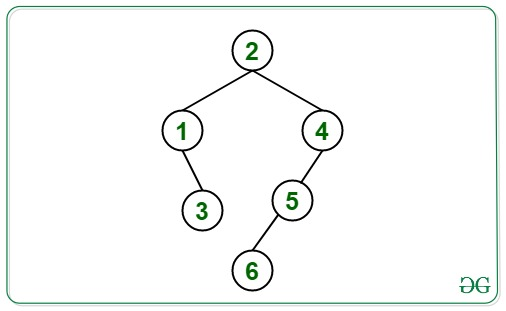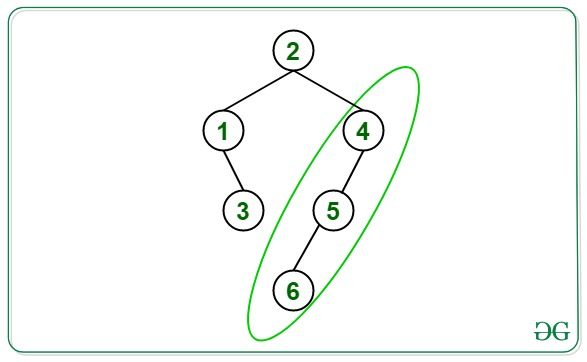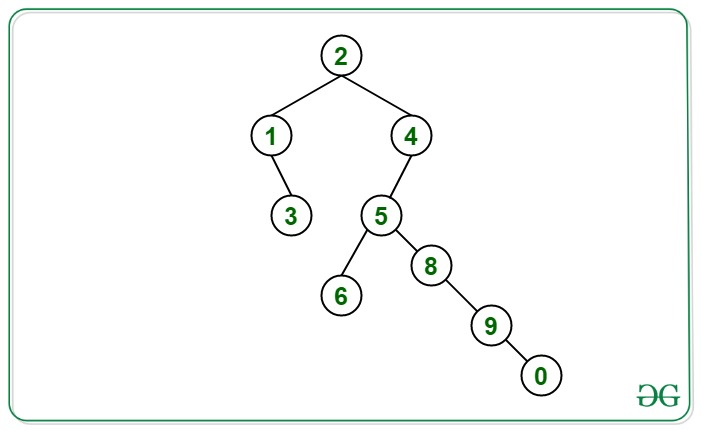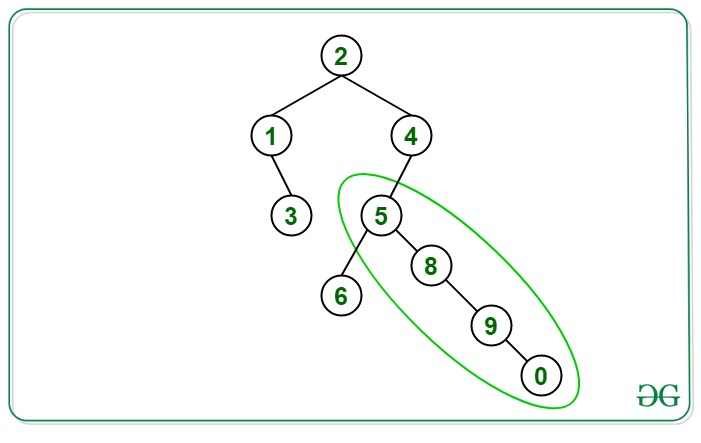Related Articles
Length of longest straight path from a given Binary Tree
• Last Updated : 03 Nov, 2020

Given a Binary Tree, the task is to find the length of the longest straight path of the given binary tree.

Straight Path is defined as the path that starts from any node and ends at another node in the tree such that the direction of traversal from the source node to the destination node always remains the same i.e., either left or right, without any change in direction that is left->left ->left or right->right->right direction.

Examples:

Input:Output: 2
Explanation:
The path shown in green is the longest straight path from 4 to 6 which is of length 2.Input:Output: 3
Explanation:
The path shown in green is the longest straight path from 5 to 0 which is of length 3.Approach: The idea is to use the Postorder traversal. Follow the steps below to solve the problem:

1. For each node, check the direction of the current Node (either left or right) and check which direction of its child is providing the longest length below it to that node.
2. If the direction of the current node and the child giving the longest length is not the same then save the result of that child and pass the length of the other child to its parent.
3. Using the above steps find the longest straight path at each node and save the result to print the maximum value among all the straight paths.
4. Print the maximum path after the above steps.

Below is the implementation of the above approach:

## C++

 `// C++ program for the above approach` `#include ``using` `namespace` `std;` `// Structure of a Tree node``struct` `Node {``    ``int` `key;``    ``struct` `Node *left, *right;``};` `// Function to create a new node``Node* newNode(``int` `key)``{``    ``Node* temp = ``new` `Node;``    ``temp->key = key;``    ``temp->left = temp->right = NULL;``    ``return` `(temp);``}` `// Function to find the longest``// straight path in a tree``int` `findPath(Node* root, ``char` `name,``             ``int``& max_v)``{``    ``// Base Case``    ``if` `(root == NULL) {``        ``return` `0;``    ``}` `    ``// Recursive call on left child``    ``int` `left = findPath(root->left,``                        ``'l'``, max_v);` `    ``// Recursive call on right child``    ``int` `right = findPath(root->right,``                         ``'r'``, max_v);` `    ``// Return the maximum straight``    ``// path possible from current node``    ``if` `(name == ``'t'``) {``        ``return` `max(left, right);``    ``}` `    ``// Leaf node``    ``if` `(left == 0 && right == 0) {``        ``return` `1;``    ``}` `    ``// Executes when either of the``    ``// child is present or both``    ``else` `{` `        ``// Pass the longest value from``        ``// either direction``        ``if` `(left < right) {``            ``if` `(name == ``'r'``)``                ``return` `1 + right;` `            ``else` `{``                ``max_v = max(max_v, right);``                ``return` `1 + left;``            ``}``        ``}``        ``else` `{``            ``if` `(name == ``'l'``)``                ``return` `1 + left;` `            ``else` `{``                ``max_v = max(max_v, left);``                ``return` `1 + right;``            ``}``        ``}``    ``}``    ``return` `0;``}` `// Driver Code``int` `main()``{` `    ``// Given Tree``    ``Node* root = newNode(3);` `    ``root->left = newNode(3);``    ``root->right = newNode(3);` `    ``root->left->right = newNode(2);``    ``root->right->left = newNode(4);``    ``root->right->left->left = newNode(4);` `    ``int` `max_v = max(``        ``findPath(root, ``'t'``, max_v),``        ``max_v);` `    ``// Print the maximum length``    ``cout << max_v << ``"\n"``;``}`

## Java

 `// Java program for the above approach``import` `java.util.*;` `class` `GFG{``    ` `static` `int` `max_v;` `// Structure of a Tree node``static` `class` `Node``{``    ``int` `key;``    ``Node left, right;``};` `// Function to create a new node``static` `Node newNode(``int` `key)``{``    ``Node temp = ``new` `Node();``    ``temp.key = key;``    ``temp.left = temp.right = ``null``;``    ``return` `(temp);``}` `// Function to find the longest``// straight path in a tree``static` `int` `findPath(Node root, ``char` `name)``{``    ` `    ``// Base Case``    ``if` `(root == ``null``)``    ``{``        ``return` `0``;``    ``}` `    ``// Recursive call on left child``    ``int` `left = findPath(root.left, ``'l'``);` `    ``// Recursive call on right child``    ``int` `right = findPath(root.right, ``'r'``);` `    ``// Return the maximum straight``    ``// path possible from current node``    ``if` `(name == ``'t'``)``    ``{``        ``return` `Math.max(left, right);``    ``}` `    ``// Leaf node``    ``if` `(left == ``0` `&& right == ``0``)``    ``{``        ``return` `1``;``    ``}` `    ``// Executes when either of the``    ``// child is present or both``    ``else``    ``{``        ` `        ``// Pass the longest value from``        ``// either direction``        ``if` `(left < right)``        ``{``            ``if` `(name == ``'r'``)``                ``return` `1` `+ right;``            ``else``            ``{``                ``max_v = Math.max(max_v, right);``                ``return` `1` `+ left;``            ``}``        ``}``        ``else``        ``{``            ``if` `(name == ``'l'``)``                ``return` `1` `+ left;``            ``else``            ``{``                ``max_v = Math.max(max_v, left);``                ``return` `1` `+ right;``            ``}``        ``}``    ``}``}` `// Driver Code``public` `static` `void` `main(String[] args)``{` `    ``// Given Tree``    ``Node root = newNode(``3``);` `    ``root.left = newNode(``3``);``    ``root.right = newNode(``3``);``    ``root.left.right = newNode(``2``);``    ``root.right.left = newNode(``4``);``    ``root.right.left.left = newNode(``4``);` `    ``max_v = Math.max(findPath(root, ``'t'``),``                     ``max_v);` `    ``// Print the maximum length``    ``System.out.print(max_v+ ``"\n"``);``}``}` `// This code is contributed by Amit Katiyar`

## Python3

 `# Python3 program for the above approach``max_v ``=` `0` `# Structure of a Tree node``class` `newNode:``    ` `    ``def` `__init__(``self``, key):``        ` `        ``self``.key ``=` `key``        ``self``.left ``=` `None``        ``self``.right ``=` `None` `# Function to find the longest``# straight path in a tree``def` `findPath(root, name):``    ` `    ``global` `max_v``    ` `    ``# Base Case``    ``if` `(root ``=``=` `None``):``        ``return` `0` `    ``# Recursive call on left child``    ``left ``=` `findPath(root.left, ``'l'``)` `    ``# Recursive call on right child``    ``right ``=` `findPath(root.right, ``'r'``)``    ` `    ``# Return the maximum straight``    ``# path possible from current node``    ``if` `(name ``=``=` `'t'``):``        ``return` `max``(left, right)` `    ``# Leaf node``    ``if` `(left ``=``=` `0` `and` `right ``=``=` `0``):``        ``return` `1` `    ``# Executes when either of the``    ``# child is present or both``    ``else``:``        ` `        ``# Pass the longest value from``        ``# either direction``        ``if` `(left < right):``            ``if` `(name ``=``=` `'r'``):``                ``return` `1` `+` `right``            ``else``:``                ``max_v ``=` `max``(max_v, right)``                ``return` `1` `+` `left``        ``else``:``            ``if` `(name ``=``=` `'l'``):``                ``return` `1` `+` `left``            ``else``:``                ``max_v ``=` `max``(max_v, left)``                ``return` `1` `+` `right``                ` `    ``return` `0` `def` `helper(root):``    ` `    ``global` `max_v``    ``temp ``=` `max``(findPath(root, ``'t'``), max_v)``    ``print``(temp)` `# Driver Code``if` `__name__ ``=``=` `'__main__'``:``    ` `    ``# Given Tree``    ``root ``=` `newNode(``3``)``    ``root.left ``=` `newNode(``3``)``    ``root.right ``=` `newNode(``3``)``    ``root.left.right ``=` `newNode(``2``)``    ``root.right.left ``=` `newNode(``4``)``    ``root.right.left.left ``=` `newNode(``4``)``    ` `    ``helper(root)` `# This code is contributed by ipg2016107`

## C#

 `// C# program for``// the above approach``using` `System;``class` `GFG{``    ` `static` `int` `max_v;` `// Structure of a Tree node``public` `class` `Node``{``  ``public` `int` `key;``  ``public` `Node left, right;``};` `// Function to create a new node``static` `Node newNode(``int` `key)``{``  ``Node temp = ``new` `Node();``  ``temp.key = key;``  ``temp.left = temp.right = ``null``;``  ``return` `(temp);``}` `// Function to find the longest``// straight path in a tree``static` `int` `findPath(Node root,``                    ``char` `name)``{``  ``// Base Case``  ``if` `(root == ``null``)``  ``{``    ``return` `0;``  ``}` `  ``// Recursive call on left child``  ``int` `left = findPath(root.left, ``'l'``);` `  ``// Recursive call on right child``  ``int` `right = findPath(root.right, ``'r'``);` `  ``// Return the maximum straight``  ``// path possible from current node``  ``if` `(name == ``'t'``)``  ``{``    ``return` `Math.Max(left, right);``  ``}` `  ``// Leaf node``  ``if` `(left == 0 && right == 0)``  ``{``    ``return` `1;``  ``}` `  ``// Executes when either of the``  ``// child is present or both``  ``else``  ``{``    ``// Pass the longest value from``    ``// either direction``    ``if` `(left < right)``    ``{``      ``if` `(name == ``'r'``)``        ``return` `1 + right;``      ``else``      ``{``        ``max_v = Math.Max(max_v, right);``        ``return` `1 + left;``      ``}``    ``}``    ``else``    ``{``      ``if` `(name == ``'l'``)``        ``return` `1 + left;``      ``else``      ``{``        ``max_v = Math.Max(max_v, left);``        ``return` `1 + right;``      ``}``    ``}``  ``}``}` `// Driver Code``public` `static` `void` `Main(String[] args)``{``  ``// Given Tree``  ``Node root = newNode(3);` `  ``root.left = newNode(3);``  ``root.right = newNode(3);``  ``root.left.right = newNode(2);``  ``root.right.left = newNode(4);``  ``root.right.left.left = newNode(4);` `  ``max_v = Math.Max(findPath(root, ``'t'``), max_v);` `  ``// Print the maximum length``  ``Console.Write(max_v + ``"\n"``);``}``}` `// This code is contributed by 29AjayKumar`
Output:
```2

```

Time Complexity: O(N)
Auxiliary Space: O(1)

My Personal Notes arrow_drop_up